## Following is my submission for Kaggle’s Titanic Competition

In :
```import pandas as pd
import numpy as np
```
In :
```df_train = pd.read_csv(r'C:UserspiushDesktopDatasetTitanictrain.csv')
```
In :
```df_train.head(2)
```
Out:
PassengerId Survived Pclass Name Sex Age SibSp Parch Ticket Fare Cabin Embarked
0 1 0 3 Braund, Mr. Owen Harris male 22.0 1 0 A/5 21171 7.2500 NaN S
1 2 1 1 Cumings, Mrs. John Bradley (Florence Briggs Th… female 38.0 1 0 PC 17599 71.2833 C85 C
In :
```df_train.info()
```
```<class 'pandas.core.frame.DataFrame'>
RangeIndex: 891 entries, 0 to 890
Data columns (total 12 columns):
PassengerId    891 non-null int64
Survived       891 non-null int64
Pclass         891 non-null int64
Name           891 non-null object
Sex            891 non-null object
Age            714 non-null float64
SibSp          891 non-null int64
Parch          891 non-null int64
Ticket         891 non-null object
Fare           891 non-null float64
Cabin          204 non-null object
Embarked       889 non-null object
dtypes: float64(2), int64(5), object(5)
memory usage: 83.6+ KB
```

So the data has 891 rows of survived, however in some columns there is some data missing Age,Cabin,Embarked, Cabin

Also, Name,Sex,Cabin,Embarked are objects and rest all are numerical data types.

We drop PassengerId, Name and Cabin as we do not need it.

In :
```df_train = df_train.drop(['Cabin','Name'],axis = 1)
```

There are a number of ways that we could fill in the NaN values of the column Age. For simplicity, we’ll do so by taking the median of values of each column.

In :
```age_median = df_train['Age'].median()
df_train['Age'] = df_train['Age'].fillna(age_median)
```

Taking the average/ median does not make sense for the column Embarked, as it is a categorical value. Instead, we shall replace the NaN values by the mode, or most frequently occurring value.

In :
```mode_embarked = df_train['Embarked'].mode().values
df_train['Embarked'] = df_train['Embarked'].fillna(mode_embarked)
```

## Converting to numerical variables

In :
```df_train['Gender'] = df_train['Sex'].map({'female': 0, 'male': 1}).astype(int)
df_train['Port'] = df_train['Embarked'].map({'C':1, 'S':2, 'Q':3}).astype(int)

df_train = df_train.drop(['Sex', 'Embarked'], axis=1)

cols = df_train.columns.tolist()
cols = [cols] + cols[0:1] + cols[2:]
df_train = df_train[cols]
```
In :
```df_train['Ticket'] = pd.get_dummies(df_train['Ticket'],prefix ='Ticket')
```
In :
```df_train.info()
```
```<class 'pandas.core.frame.DataFrame'>
RangeIndex: 891 entries, 0 to 890
Data columns (total 10 columns):
Survived       891 non-null int64
PassengerId    891 non-null int64
Pclass         891 non-null int64
Age            891 non-null float64
SibSp          891 non-null int64
Parch          891 non-null int64
Ticket         891 non-null float64
Fare           891 non-null float64
Gender         891 non-null int32
Port           891 non-null int32
dtypes: float64(3), int32(2), int64(5)
memory usage: 62.7 KB
```

Hence have we have preserved all the rows of our data set, and proceed to create a numerical array for Scikit-learn.

In :
```#print summary of data frame
summary = df_train.describe()
print(summary)
```
```         Survived  PassengerId      Pclass         Age       SibSp
count  891.000000   891.000000  891.000000  891.000000  891.000000
mean     0.383838   446.000000    2.308642   29.361582    0.523008
std      0.486592   257.353842    0.836071   13.019697    1.102743
min      0.000000     1.000000    1.000000    0.420000    0.000000
25%      0.000000   223.500000    2.000000   22.000000    0.000000
50%      0.000000   446.000000    3.000000   28.000000    0.000000
75%      1.000000   668.500000    3.000000   35.000000    1.000000
max      1.000000   891.000000    3.000000   80.000000    8.000000

Parch      Ticket        Fare      Gender        Port
count  891.000000  891.000000  891.000000  891.000000  891.000000
mean     0.381594    0.003367   32.204208    0.647587    1.897868
std      0.806057    0.057961   49.693429    0.477990    0.514624
min      0.000000    0.000000    0.000000    0.000000    1.000000
25%      0.000000    0.000000    7.910400    0.000000    2.000000
50%      0.000000    0.000000   14.454200    1.000000    2.000000
75%      0.000000    0.000000   31.000000    1.000000    2.000000
max      6.000000    1.000000  512.329200    1.000000    3.000000
```
In :
```df_train.columns
```
Out:
```Index(['Survived', 'PassengerId', 'Pclass', 'Age', 'SibSp', 'Parch', 'Ticket',
'Fare', 'Gender', 'Port'],
dtype='object')```

# Visualizing

### Parallel Coordinates Plots

In :
```import matplotlib.pyplot as plot
%matplotlib inline

for i in range(891):
#assign color based on survived based on "0" or "1" labels
if df_train.iat[i,8] == 1:
pcolor = "red"
else:
pcolor = "blue"

#plot rows of data as if they were series data
dataRow = df_train.iloc[i,0:8]
dataRow.plot(color=pcolor, alpha=0.5)

plot.xlabel("Attribute Index")
plot.ylabel(("Attribute Values"))
plot.show()
```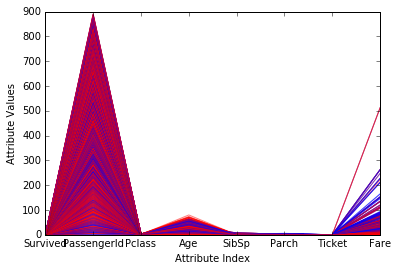Fare seems to stand out the most. Could be a factor to look at in the future for survival

### Plotting a scatter plot between the survival and attribute Fare.

In :
```target = df_train['Survived']
#plot rows of data as if they were series data
dataRow = df_train.iloc[0:891,6]
plot.scatter(dataRow, target,alpha=0.5, s=120)

plot.xlabel("Attribute Value")
plot.ylabel("Target Value")
plot.show()
```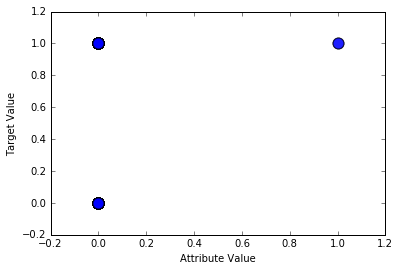This doesnot provide much help to see if fare was a factor in survival.

In :
```#calculate correlations between attributes

corMat = pd.DataFrame(df_train.corr())

#visualize correlations using heatmap
plot.pcolor(corMat)
plot.show()
```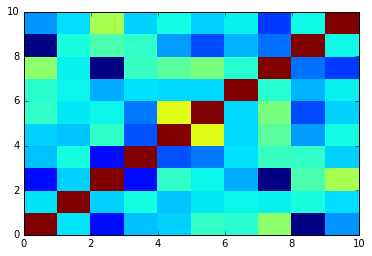In :
```labels = df_train['Survived'].values
df_train1 = df_train[['PassengerId','Pclass','Age', 'SibSp', 'Parch', 'Ticket', 'Fare','Gender', 'Port']]
xList = df_train1.values

```
In :
```from sklearn.ensemble import RandomForestClassifier

clf = RandomForestClassifier(n_estimators=100)
```

Fit the training data and create the decision trees:

In :
```# Training data features, skip the first column 'Survived'
train_features = xList[:, 1:]

# 'Survived' column values
train_target = labels

# Fit the model to our training data
clf = clf.fit(train_features, train_target)
score = clf.score(train_features, train_target)
"Mean accuracy of Random Forest: {0}".format(score)
```
Out:
`'Mean accuracy of Random Forest: 0.9797979797979798'`
In :
```#import random
from sklearn import datasets, linear_model

def confusionMatrix(predicted, actual, threshold):
if len(predicted) != len(actual): return -1
tp = 0.0
fp = 0.0
tn = 0.0
fn = 0.0
for i in range(len(actual)):
if actual[i] > 0.5: #labels that are 1.0  (positive examples)
if predicted[i] > threshold:
tp += 1.0 #correctly predicted positive
else:
fn += 1.0 #incorrectly predicted negative
else:              #labels that are 0.0 (negative examples)
if predicted[i] < threshold:
tn += 1.0 #correctly predicted negative
else:
fp += 1.0 #incorrectly predicted positive
rtn = [tp, fn, fp, tn]
return rtn

#divide attribute matrix and label vector into training(2/3 of data) and test sets (1/3 of data)
indices = range(len(xList))
xListTest = [xList[i] for i in indices if i%3 == 0 ]
xListTrain = [xList[i] for i in indices if i%3 != 0 ]
labelsTest = [labels[i] for i in indices if i%3 == 0]
labelsTrain = [labels[i] for i in indices if i%3 != 0]

#form list of list input into numpy arrays to match input class for scikit-learn linear model
xTrain = np.array(xListTrain); yTrain = np.array(labelsTrain); xTest = np.array(xListTest); yTest = np.array(labelsTest)

#check shapes to see what they look like
print("Shape of xTrain array", xTrain.shape)
print("Shape of yTrain array", yTrain.shape)
print("Shape of xTest array", xTest.shape)
print("Shape of yTest array", yTest.shape)
```
```Shape of xTrain array (594, 9)
Shape of yTrain array (594,)
Shape of xTest array (297, 9)
Shape of yTest array (297,)
```
In :
```#train linear regression model
dfModel = linear_model.LinearRegression()
dfModel.fit(xTrain,yTrain)

#generate predictions on in-sample error
trainingPredictions = dfModel.predict(xTrain)
print("Some values predicted by model", trainingPredictions[0:5], trainingPredictions[-6:-1])
```
```Some values predicted by model [ 0.9323192   0.68526999  0.05711893  0.07343276  0.08090763] [ 0.27789897  0.12976048  0.28570535  1.08136189  0.49732495]
```
In :
```#generate confusion matrix for predictions on training set (in-sample
confusionMatTrain = confusionMatrix(trainingPredictions, yTrain, 0.50)
#pick threshold value and generate confusion matrix entries
tp = confusionMatTrain; fn = confusionMatTrain; fp = confusionMatTrain; tn = confusionMatTrain

print("tp = " + str(tp) + "tfn = " + str(fn) + "n" + "fp = " + str(fp) + "ttn = " + str(tn) + 'n')

#Misclassification error rate
def errorRate(tp,fn,fp,tn):
#Accuracy
acc = (tp + tn) / (tp + fn + fp + tn)
return 1- acc

print("Miscalssification error rate is : " + str(errorRate(tp,fn,fp,tn)))
```
```tp = 161.0	fn = 68.0
fp = 42.0	tn = 323.0

Miscalssification error rate is : 0.18518518518518523
```
In :
```#generate predictions on out-of-sample data
testPredictions = dfModel.predict(xTest)

#generate confusion matrix from predictions on out-of-sample data
conMatTest = confusionMatrix(testPredictions, yTest, 0.25)
#pick threshold value and generate confusion matrix entries
tp = conMatTest; fn = conMatTest; fp = conMatTest; tn = conMatTest
print("tp = " + str(tp) + "tfn = " + str(fn) + "n" + "fp = " + str(fp) + "ttn = " + str(tn) + 'n')

print("Miscalssification error rate is : " + str(errorRate(tp,fn,fp,tn)))
```
```tp = 89.0	fn = 24.0
fp = 67.0	tn = 117.0

Miscalssification error rate is : 0.30639730639730645
```
In :
```from sklearn.metrics import roc_curve, auc
#generate ROC curve for in-sample

fpr, tpr, thresholds = roc_curve(yTrain,trainingPredictions)
roc_auc = auc(fpr, tpr)
print( 'AUC for in-sample ROC curve: %f' % roc_auc)
```
```AUC for in-sample ROC curve: 0.877897
```
In :
```from sklearn.cross_validation import train_test_split
from sklearn import ensemble
from sklearn.metrics import roc_auc_score

#number of rows and columns in x matrix
nrows = len(xList)
ncols = len(xList)

#form x and y into numpy arrays and make up column names
X = np.array(xList)
y = np.array(labels)
dfNames = np.array(['V' + str(i) for i in range(ncols)])

#break into training and test sets.
xTrain, xTest, yTrain, yTest = train_test_split(X, y, test_size=0.30, random_state=531)

auc = []

nTreeList = range(50, 2000, 50)
for iTrees in nTreeList:
depth = None
maxFeat  = 8 #try tweaking
dfRFModel = ensemble.RandomForestClassifier(n_estimators=iTrees, max_depth=depth, max_features=maxFeat,
oob_score=False, random_state=531)

dfRFModel.fit(xTrain,yTrain)

#Accumulate auc on test set
prediction = dfRFModel.predict_proba(xTest)
aucCalc = roc_auc_score(yTest, prediction[:,1:2])
auc.append(aucCalc)

print("AUC" )
print(auc[-1])
```
```AUC
0.869512824927
```
In :
```#plot training and test errors vs number of trees in ensemble
plot.plot(nTreeList, auc)
plot.xlabel('Number of Trees in Ensemble')
plot.ylabel('Area Under ROC Curve - AUC')
#plot.ylim([0.0, 1.1*max(mseOob)])
plot.show()
```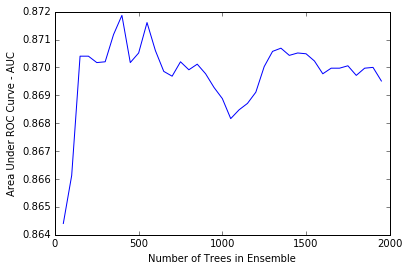In :
```df_train1.columns
```
Out:
```Index(['PassengerId', 'Pclass', 'Age', 'SibSp', 'Parch', 'Ticket', 'Fare',
'Gender', 'Port'],
dtype='object')```
In :
```# Plot feature importance
featureImportance = dfRFModel.feature_importances_
# normalize by max importance
featureImportance = featureImportance / featureImportance.max()

#plot importance of 8
idxSorted = np.argsort(featureImportance)[0:9]
idxTemp = np.argsort(featureImportance)[::-1]
print(idxTemp)
barPos = np.arange(idxSorted.shape) + .5
plot.barh(barPos, featureImportance[idxSorted], align='center')
plot.yticks(barPos, dfNames[idxSorted])
plot.xlabel('Variable Importance')
plot.show()
```
```[7 0 6 2 1 3 8 4 5]
```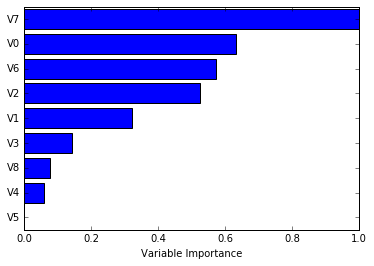In :
```#plot best version of ROC curve
fpr, tpr, thresh = roc_curve(yTest, list(prediction[:,1:2]))
ctClass = [i*0.01 for i in range(101)]

plot.plot(fpr, tpr, linewidth=2)
plot.plot(ctClass, ctClass, linestyle=':')
plot.xlabel('False Positive Rate')
plot.ylabel('True Positive Rate')
plot.show()
```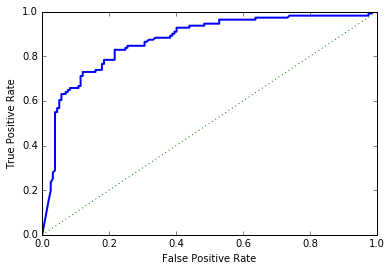In :
```#form x and y into numpy arrays and make up column names
X = np.array(df_train1)
y = np.array(labels)
dfNames = np.array(['V' + str(i) for i in range(ncols)])

#break into training and test sets.
xTrain, xTest, yTrain, yTest = train_test_split(X, y, test_size=0.30, random_state=531)
#instantiate model
nEst = 2000
depth = 3
learnRate = 0.007
maxFeatures = 8
learning_rate=learnRate,
max_features=maxFeatures)
#train
dfGBMModel.fit(xTrain, yTrain)

# compute auc on test set as function of ensemble size
auc = []
aucBest = 0.0
predictions = dfGBMModel.staged_decision_function(xTest)
for p in predictions:
aucCalc = roc_auc_score(yTest, p)
auc.append(aucCalc)

#capture best predictions
if aucCalc > aucBest:
aucBest = aucCalc
pBest = p

idxBest = auc.index(max(auc))

#print best values
print("Best AUC" )
print(auc[idxBest])
print("Number of Trees for Best AUC")
print(idxBest)
```
```Best AUC
0.871922878292
Number of Trees for Best AUC
1127
```
In :
```df_test = pd.read_csv(r'C:UserspiushDesktopDatasetTitanictest.csv')
```
In :
```df_test.head(2)
```
Out:
PassengerId Pclass Name Sex Age SibSp Parch Ticket Fare Cabin Embarked
0 892 3 Kelly, Mr. James male 34.5 0 0 330911 7.8292 NaN Q
1 893 3 Wilkes, Mrs. James (Ellen Needs) female 47.0 1 0 363272 7.0000 NaN S
In :
```df_test.info()
```
```<class 'pandas.core.frame.DataFrame'>
RangeIndex: 418 entries, 0 to 417
Data columns (total 11 columns):
PassengerId    418 non-null int64
Pclass         418 non-null int64
Name           418 non-null object
Sex            418 non-null object
Age            332 non-null float64
SibSp          418 non-null int64
Parch          418 non-null int64
Ticket         418 non-null object
Fare           417 non-null float64
Cabin          91 non-null object
Embarked       418 non-null object
dtypes: float64(2), int64(4), object(5)
memory usage: 36.0+ KB
```
In :
```df_test = df_test.drop(['Cabin','Name'],axis = 1)
```
In :
```age_median = df_test['Age'].median()
df_test['Age'] = df_test['Age'].fillna(age_median)
```
In :
```mean_fare = df_test['Fare'].mean()
df_test['Fare'] = df_test['Fare'].fillna(mean_fare)
```
In :
```df_test['Gender'] = df_test['Sex'].map({'female': 0, 'male': 1}).astype(int)
df_test['Port'] = df_test['Embarked'].map({'C':1, 'S':2, 'Q':3}).astype(int)

df_test = df_test.drop(['Sex', 'Embarked'], axis=1)

cols = df_test.columns.tolist()
cols = [cols] + cols[0:1] + cols[2:]
df_test = df_test[cols]
```
In :
```df_test['Ticket'] = pd.get_dummies(df_test['Ticket'],prefix ='Ticket')
```
In :
```df_test.info()
```
```<class 'pandas.core.frame.DataFrame'>
RangeIndex: 418 entries, 0 to 417
Data columns (total 9 columns):
Pclass         418 non-null int64
PassengerId    418 non-null int64
Age            418 non-null float64
SibSp          418 non-null int64
Parch          418 non-null int64
Ticket         418 non-null float64
Fare           418 non-null float64
Gender         418 non-null int32
Port           418 non-null int32
dtypes: float64(3), int32(2), int64(4)
memory usage: 26.2 KB
```
In :
```# Data wrangle the test set and convert it to a numpy array
test_data = df_test.values
```

Take the decision trees and run it on the test data:

In :
```# Get the test data features, skipping the first column 'PassengerId'
test_x = test_data[:,]

# Predict the Survival values for the test data
test_y =list(map(int, dfRFModel.predict(test_x)))
```

# Random Forest: Prepare for Kaggle Submission

Create a DataFrame by combining the index from the test data with the output of predictions, then write the results to the output:

In :
```df_test['Survived'] = test_y
df_test[['PassengerId', 'Survived']]
.to_csv('results-3rf.csv', index=False)```

### This Post Has One Comment

1. Anwar

Good job. What was the LB score?

This site uses Akismet to reduce spam. Learn how your comment data is processed.